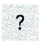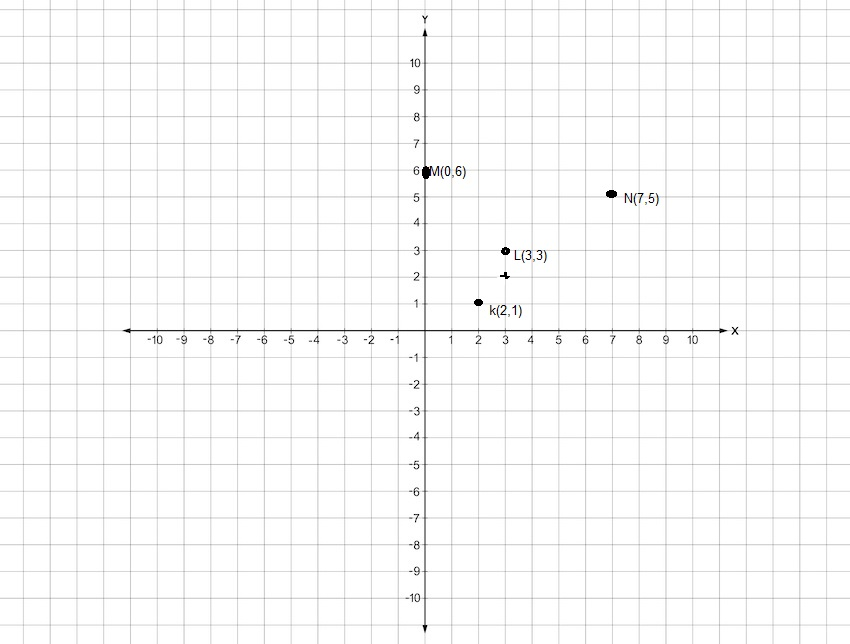# Math in Focus Grade 6 Chapter 8 Answer Key Equations and Inequalities

Practice the problems of Math in Focus Grade 6 Workbook Answer Key Chapter 8 Equations and Inequalities to score better marks in the exam.

## Math in Focus Grade 6 Course 1 B Chapter 8 Answer Key Multiplying and Dividing Fractions and Decimals

### Math in Focus Grade 6 Chapter 8 Quick Check Answer Key

Complete with =, >, or <.

Question 1.
25-26
25 > -26,

Explanation:
Given to compare 25 and -26 as 25 is greater than -26 therefore 25 > -26.

Question 2.
12 + 12 + 123 ∙ 12
12 + 12 + 12 = 36,

Explanation:
As 12 + 12 + 12 = 36 and 3 X 12 is 36 so both are equal therefore 12  + 12 + 12 = 36.

Question 3.
40 ÷ 88 ÷ 40
40 ÷ 8 = 8 ÷ 40,

Explanation:
As 40 ÷ 8 is 5 and 8 ÷ 40 is 5 therefore 40 ÷ 8 = 8 ÷ 40.

Question 4.
-16-7
-16 < -7,

Explanation:
Given to compare between – 16 and – 7 as – 16 is lessthan -7 so -16 < -7.

Write an algebraic expression for each of the following.

Question 5.
The sum of 15 and p
x = 15 + p,

Explanation:
An algebraic expression for the sum of 15 and p, let it be x so x = 15 + p.

Question 6.
The difference “q less than 10”
x – q < 10,

Explanation:
Given the difference “q less than 10” is let it be x so  x – q < 10.

Question 7.
The product of r and 23
x = r X 23,

Explanation:
Given the product of r and 23 is let it be x so x = r X 23.

Question 8.
Divide s by 11.
x = s ÷ 11,

Explanation:
Given divide s by 11 so let it be x so x = s ÷ 11.

Evaluate each expression for the given values of the variable.

Question 9.
3x + 5 when x = 9 and x = 12
3x + 5 = 32, when x = 9,
3x + 5 = 41, when x = 12,

Explanation:
Given to find 3x + 5 when x = 9, so 3 X 9 + 5 = 27 + 5 = 32, when x = 12 we get 3x + 5 = 3 x 12 + 5 = 36 + 5 = 41.

Question 10.
28 – 4x when x = 4 and x = 7
28 – 4x = 12, when x = 4,
28 – 4x = 0 when x = 7,

Explanation:
Given when x = 4 the equation 28 – 4x is 28 – 16 = 12, when x = 7 then the equation becomes 28 – 4 X 7 = 28 – 28 = 0.

Plot the points on a coordinate plane.

Question 11.
K (2, 1), L (3, 3), M (0, 6), and N (7, 5)